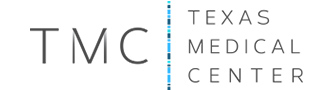# Our Clients

A big thanks to our wonderful clients! A sampling of the groups we work with listed here.

` ` ` `

` `

` `` `

` `` `

• ` ` ` `
` ` ` ` ` `` `` `` ` ` ` ` `

` ` ` ` ` `

### Astros Foundation

` `` `` `

` `` `` `

` `` `

` `

` `

` ` ` `` ` ` `

` `

` `` `

` `` `

• ` ` ` `
` ` ` ` ` `` `` `` ` ` ` ` `

` ` ` ` ` `

### Simon

` `` `` `

` `` `` `

` `` `

` `

` `

` ` ` `` ` ` `

` `

` `` `

` `` `

• ` ` ` `
` ` ` ` ` `` `` `` ` ` ` ` `

` ` ` ` ` `

### Gensler

` `

` `

` ` ` `` ` ` `

` `

` `` `

` `` `

• ` ` ` `
` ` ` ` ` `` `` `` ` ` ` ` `

` ` ` ` ` `

### Neiman Marcus

` `

` `

` ` ` `` ` ` `

` `

` `` `

` `` `

• ` ` ` `
` ` ` ` ` `` `` `` ` ` ` ` `

` ` ` ` ` `

### Texas Medical Center

` `` `` `

` `` `` `

` `` `

` `

` `

` ` ` `` ` ` `

` `

` `` `

` `` `

• ` ` ` `
` ` ` ` ` `` `` `` ` ` ` ` `

` ` ` ` ` `

### River Oaks Country Club

` `

` `

` ` ` `` ` ` `

` `

` `` `

` `` `

• ` ` ` `
` ` ` ` ` `` `` `` ` ` ` ` `

` ` ` ` ` `

### Belle of the Ball

` `` `` `

` `` `` `

` `` `

` `

` `

` ` ` `` ` ` `` `` `

` `` `

• ` ` ` `
` ` ` ` ` `` `` `` ` ` ` ` `

` ` ` ` ` `

### Keely Thorne Events

` `` `` `

` `` `` `

` `` `

` `

` ` ` `` `` `` `

• ` ` ` ` ` ` ` `
` ` ` ` ` ` ` ` ` `` `` `` `` ` ` ` ` ` ` ` ` `

` ` ` ` ` ` ` ` ` `

### Houston Ballet

` `` `` `` `

` `` `` `` `

` `` `` `

` `` `` `` `

• ` ` ` ` ` ` ` `
` ` ` ` ` ` ` ` ` `` `` `` `` ` ` ` ` ` ` ` ` `

` ` ` ` ` ` ` ` ` `

### City Kitchen Catering

` `` `` `` `

` `` `` `` `

` `` `` `

` `` `` `` `

• ` ` ` ` ` ` ` `
` ` ` ` ` ` ` ` ` `` `` `` `` ` ` ` ` ` ` ` ` `

` ` ` ` ` ` ` ` ` `

### Discovery Green

` `` `` `` `

` `` `` `` `

` `` `` `

` `` `` `` `

• ` ` ` ` ` ` ` `
` ` ` ` ` ` ` ` ` `` `` `` `` ` ` ` ` ` ` ` ` `

` ` ` ` ` ` ` ` ` `

### Jackson & Company

` `` `` `` `

` `` `` `` `

` `` `` `

` `` `` `` `

• ` ` ` ` ` ` ` `
` ` ` ` ` ` ` ` ` `` `` `` `` ` ` ` ` ` ` ` ` `

` ` ` ` ` ` ` ` ` `

### Houston Magazine

` `` `` `` `

` `` `` `` `

` `` `` `